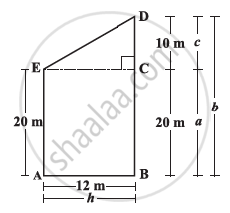Share

# Area of Trapezium

#### notesArea of  triangle ECD = 1/2 h × c = 1/2 x 12 x10 = 60 sq m.

Area of rectangle ABCE = h × a = 12 × 20 = 240 sq m

Area of trapezium ABDE = area of triangle ECD + Area of rectangle ABCE = 60 + 240 = 300 sq m

We can write the area by combining the two areas and write the A(trapezium)

area of ABDE = 1/2 h x c+ h x a

= h(c/2+a)

=h(c+(2a)/2)

=h(c+a+a/2)

=(h(b+a))/2

= ("height" ("sum of parallel sides"))/2

by substituting the values of h,b and a in this expression, we find (h(b+a))/2 =300 sq.m

### Shaalaa.com

Area of Trapezium [00:01:08]
S
0%

S